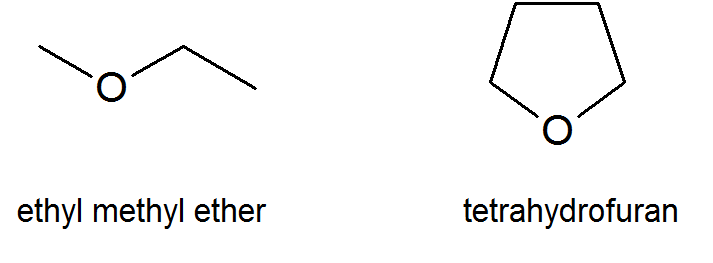# 18.0: Introduction

##### Objectives

After completing this section, you should be able to use the terms “ether,” “diethyl ether ” and “ethyl ether ” appropriately in context.

##### Key Terms

Make certain that you can define, and use in context, the key terms below.

• ether ($$\ce{R-O-R′}$$)
• sulfide ($$\ce{R-S-R′}$$)
• thiol ($$\ce{R-S-H}$$)
##### Study Notes

As defined in the textbook, an “ether” is a substance with the general formula ($$\ce{R-O-R′}$$) where $$\ce{R}$$ and $$\ce{R′}$$ are alkyl, aryl, vinyl or allyl groups. However, the word “ether” is also commonly used to refer to the specific compound, $$\ce{CH3-CH2-O-CH2-CH3}$$, which is correctly called “diethyl ether.” Further confusion can arise because some chemists refer to “diethyl ether ” as “ethyl ether.” In this course, “ether ” will be used to refer to the class of compounds with the structure ($$\ce{R-O-R′}$$); diethyl ether will refer to the compound, $$\ce{CH3-CH2-O-CH2-CH3}$$; and “ethyl ether ” will not be used.

## Ethers and Epoxides

While the general formula for ethers is R-O-R′, keep in mind that there also cyclic ethers like tetrahydrofuran (a common organic solvent) or even epoxides which you first encounter in Section 8.7 in synthesizing diols from alkenes.## Thiols and Sulfides

Thiols (thio alcohols or mercaptans) and sulfides (thioethers) are the sulfur analogues of alcohols and ethers and have the general formulas of R-S-H and R-S-R′, respectively.